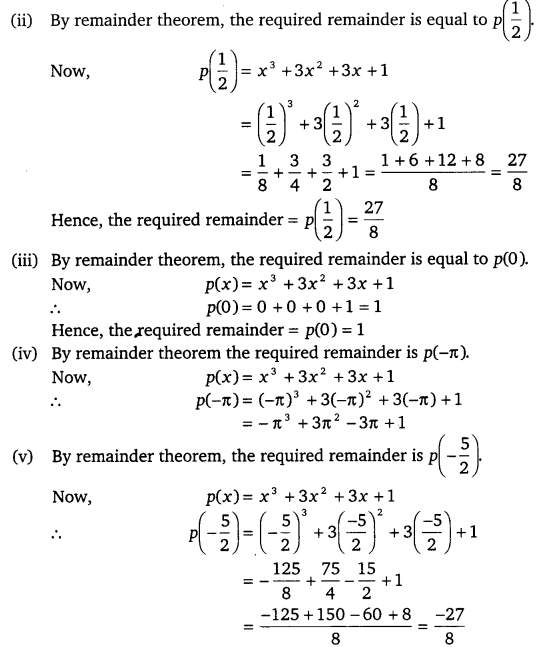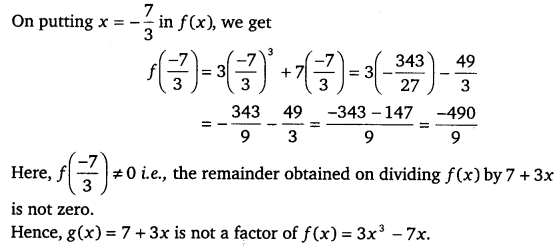# CLASS 9 MATH NCERT SOLUTION FOR CHAPTER – 2 POLYNOMIALS EX – 2.3

## Polynomials

Question 1.
Find the remainder when x3 + 3x2 + 3x + 1 is divided by
(i) x + 1
(ii) x-$\frac { 1 }{ 2 }$
(iii) x
(iv) x+π
(v) 5+2x

Solution:
(1) By remainder theorem, the required remainder is equal to p(-1).
Now, p(x)= x3+3x2+3x+1
p(-1) = (-1)3 + 3(-1)2 + 3(-1) +1 =-1+3-3+1=0
Hence, the required remainder = p(-1) = 0Question 2.
Find the remainder when x3 – ax2 + 6x – a is divided by x – a.

Solution:
Let p(x) = x3-ax2+6x-a
By remainder theorem, when p(x) is divided by x – a,
Then,remainder = p(a)
p(a)= a3-a . a2+6a-a
=a3 – a3 +6a-a = 5a

Question 3.
Check, whether 7 + 3x is a factor of 3x3 + 7x.

Solution:
Let f(x) = 3x3 + 7x and g(x) = 7 + 3x
On putting g(x) = 0, we get
7 + 3x = 0 3x = – 7
=> x =$\frac { -7 }{ 3 }$
Thus, zero of g(x) is x =$\frac { -7 }{ 3 }$.#### CLASS 9 MATH NCERT SOLUTION FOR CHAPTER – 2 POLYNOMIALS EX – 2.4

Lorem ipsum dolor sit amet, consectetur adipiscing elit. Phasellus cursus rutrum est nec suscipit. Ut et ultrices nisi. Vivamus id nisl ligula. Nulla sed iaculis ipsum.

Company Name